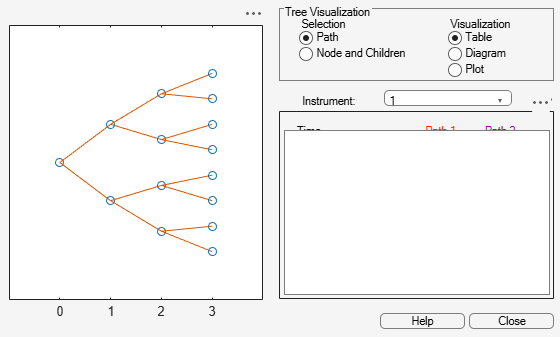# mkbush

Create bushy tree

## Syntax

``[Tree,NumStates = mkbush(NumLevels,NumChild,NumPos)``
``[Tree,NumStates = mkbush(___,Trim,NodeVal)``

## Description

example

````[Tree,NumStates = mkbush(NumLevels,NumChild,NumPos)` creates a bushy tree `Tree` with initial values `NodeVal` at each node.```

example

````[Tree,NumStates = mkbush(___,Trim,NodeVal)` adds optional arguments for `Trim` and `NodeVal`.```

## Examples

collapse all

This example shows how to create a tree with four time levels, two branches per node, and a vector of three elements in each node with each element initialized to `NaN`.

```Tree = mkbush(4, 2, 3); treeviewer(Tree) ```## Input Arguments

collapse all

Number of time levels of the tree, specified as a scalar numeric.

Data Types: `double`

Number of branches (children) of the nodes in each level, specified as a `1`-by- number of levels (`NUMLEVELS`) vector.

Data Types: `double`

Length of the state vectors in each time level, specified as a `1`-by-`NUMLEVELS` vector.

Data Types: `double`

(Optional) Indicates movement between nodes, specified as a scalar `0` or `1`. If ```Trim = 1```, `NumPos` decreases by `1` when moving from one time level to the next. Otherwise, if `Trim = 0`, `NumPos` does not decrease.

Data Types: `logical`

(Optional) Initial value at each node of the tree, specified as a scalar numeric.

Data Types: `double`

## Output Arguments

collapse all

Bushy tree, returned as a tree struct with initial values `NodeVal` at each node.

Number of state vectors in each level, returned as a `1`-by-`NUMLEVELS` vector.

## Version History

Introduced before R2006a